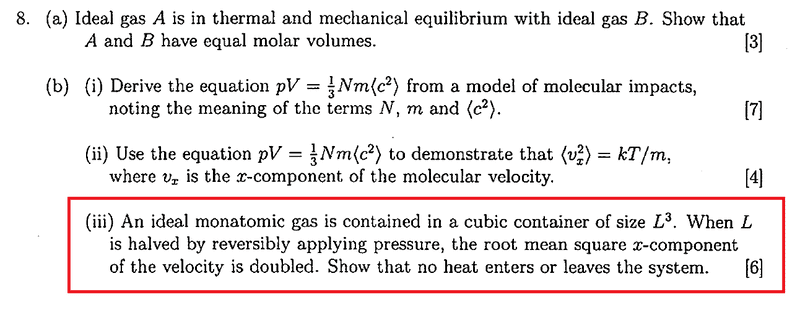## Homework Statement## The Attempt at a Solution

How to do part biii?
I tried to find change of U and W, then use ΔU-W=Q=0.
I can find the change in U by using the fact the rms of the x-component of the velocity is doubled. Therefore, originally, if isotropic <c2> = <vx2>+<vy2>+<vz2> = 3<v2x>,
now the new <vx2> is 4 times the old one, so the new mean square <c'2>=6<vx2>=2<c2>, so the new internal energy is double the old one. How about the work done? How to find it? I have only learnt how to find adiabatic, isothermal and constant volume work done.

#### Attachments

Homework Helper
Gold Member
2020 Award
If ## <v_x^2>_f=4 <v_x^2>_i ## , then ## <c^2>_f=4 <c^2>_i ##. ## \\ ## This one is hypothesized to be an adiabatic compression. You should be able to use the formula ## P_iV_i^{\gamma}=P_f V_f^{\gamma} ## to get ## P_f ## , and from that you can compute ## T_f ## on terms of ## T_i ##. If the hypothesis is correct, ## T_f=4 T_i ##. See if that is what you get.

•Clara Chung
TSny
Homework Helper
Gold Member
now the new <vx2> is 4 times the old one, so the new mean square <c'2>=6<vx2>
The factor of 6 is not correct. Check this.

Some things that might be helpful:
(1)By what factor does V change?
(2)By what factor does P change?

Are these changes compatible with an adiabatic process of a monatomic ideal gas?

I think that part (iii) of the question is poorly worded. All you are given is information about the initial and final states of the gas and the fact that the process was reversible. But between any two given states, there are infinitely many reversible processes that can take the system between the two states. These processes will generally differ in the amount of work done and the amount of heat transferred. I suspect that you are meant to show that it is possible for the process to be adiabatic. But there is not enough information to conclude that the process must have been adiabatic.

•V decreases by a factor of 8.
So if I assume the process is adiabatic,
Tf = Ti (Vi/Vf)2/3 = 4Ti
It matches Uf/Ui = <c'2>/<c2> = 4
Therefore, the hypothesis might be correct, there is no heat flow?...

••